Constructive Solid Geometry with POV-Ray

By Xah Lee. Date:

This page is a short example of POV-Ray's Constructive Solid Geometry feature. If you don't understand it, please see Intro to POV-Ray.

Intersection

POV-Ray has a feature that allows you to construct objects by specifying the intersection of other objects. For example, suppose you want to construct the object that is the intersection of two cylinders. Here's a example.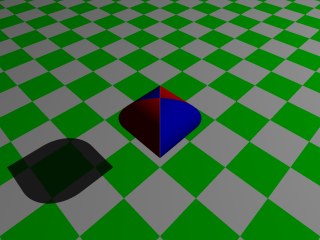The shape that is the intersection of two cylinders. 〔csg-2-cylinders.pov 〕

The key to create a intersection, is the instersection {} construct. For example, the intersection of two cylinders is specified like this:

intersection {
cylinder { <3,0,0>,<-3,0,0>, // center of two ends
texture { pigment { color Red } }
}
cylinder { <0,0,3>,<0,0,-3>, // center of two ends
texture { pigment { color Blue } }
}
}

Now, let's make a intersection of 3 orthogonal cylinders.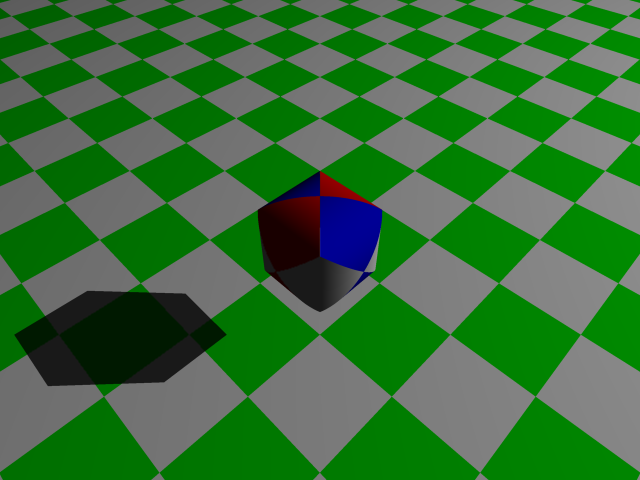A solid made by the intersection of 3 orthogonal cylinders. In such a figure, the object would have a perfect circular outline if looked on from each of its side. 〔csg-3-cylinders.pov 〕

Difference

Objects can be build by taking the difference between two objects.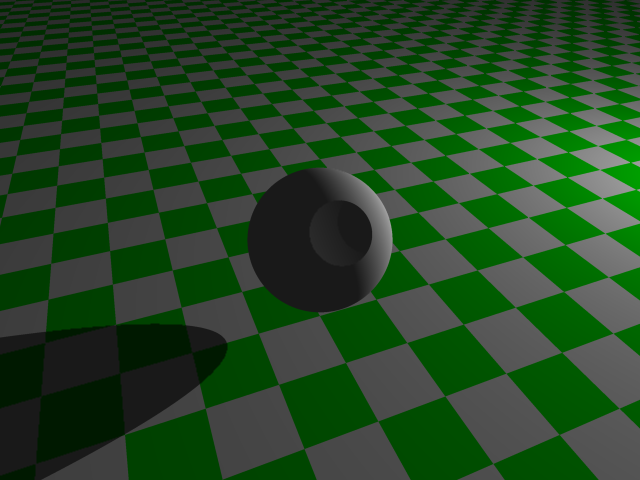A sphere with a part gouged out by a much larger sphere. 〔csg-death-star.pov 〕

The key in the above image is this:

difference {
sphere { <0,0,0>, 1}   // smaller sphere
sphere { <6,0,0>, 5.1} // larger sphere
rotate <0,0,40>        // rotate the final object for better view
pigment { White}       // color it white
}

Union and Merge

A object can be created as the union of two or more objects. Such union is useful because it can be assigned to a variable and POV-Ray can then treat it as a single object.

Here's a example.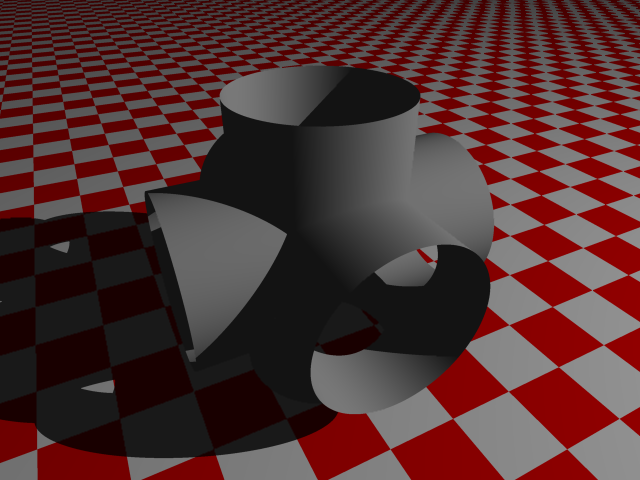The “merge” of 3 orthogonal cylinders. 〔csg-cross-pipe.pov 〕

The key for the above is this:

merge {
cylinder { <2,0,0>,<-2,0,0>, 1.1 open}
cylinder { <0,0,2>,<0,0,-2>, 1.1 open}
cylinder { <0,2,0>,<0,-2,0>, 1.1 open}
pigment { Gray }
}

Note the construction merge {}. The keyword “merge” can be replaced with “union”. Their difference is that “merge” will delete the internally shared parts. That is why, in the above image we can see thru one of the tube into another tube.

CSG Substraction Glitch

Sometimes when doing a substraction and the two object's face coincide, output image may have a glitch, because coincident surfaces confuses it. Here's a example where large square of side length 2 is to substract a smaller square of side length 1.

difference {
box { -1, 1 pigment { Red } } // -1 means <-1,-1,-1> and 1 means <1,1,1>
box { 0, 1 pigment { Blue } }
}

A proper solution, is to make the subtracted object slightly larger. For example:

difference {
box { -1, 1 pigment { Red } }
box { 0, 1 pigment { Blue } scale 1.0001}
}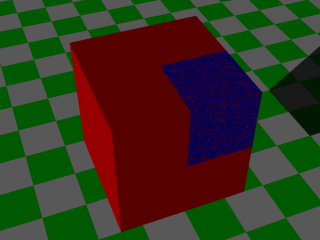Left: A CSG difference where the object's faces coincide and confuses POV-Ray. Right: The subtracted object made larger. 〔csg-diff-cube2.pov 〕

If you have a question, put \$5 at patreon and message me.